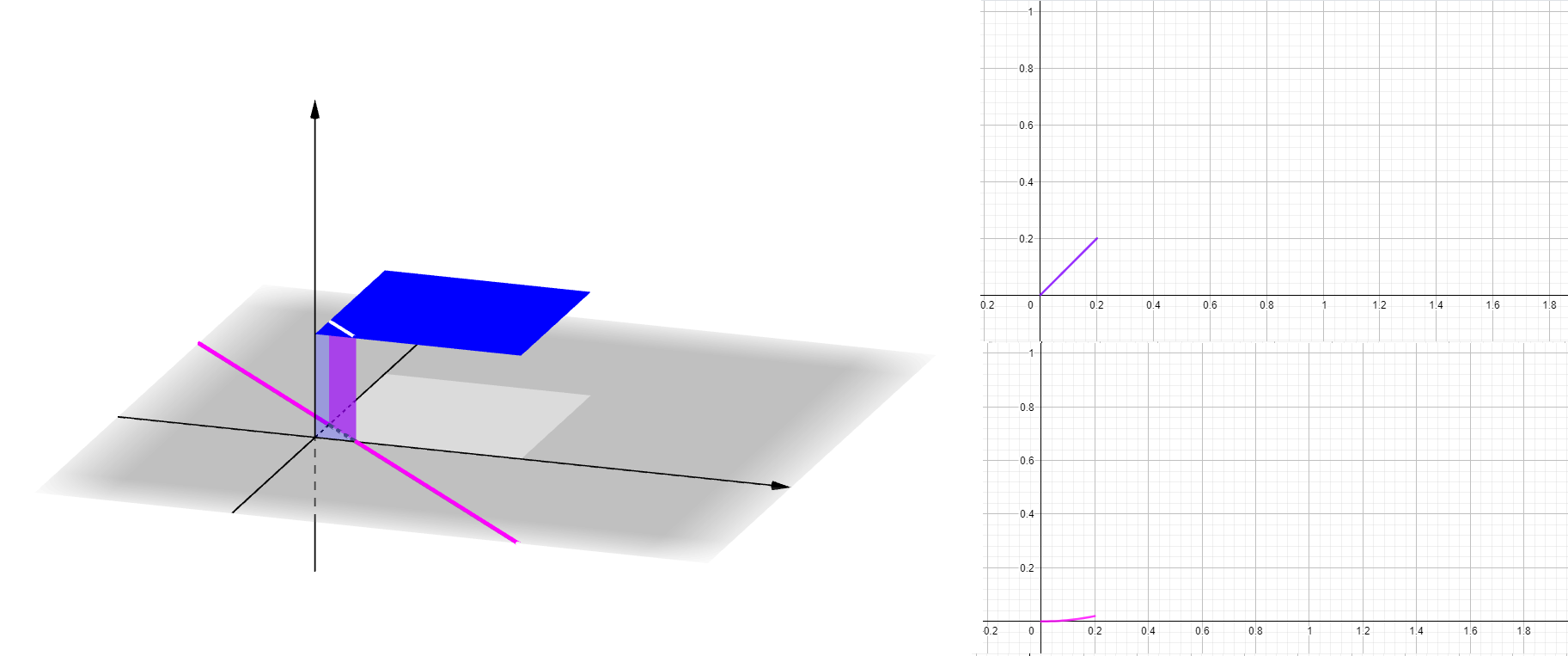# How can insert two 2D plots of single variable functions f(x) on a 3D Geogebra document?

antoni.parellada shared this question 2 years ago

I would like to insert functions of the variable c in the Slider, corresponding to the pdf and the cdf of the random variable Z = X + Y, where X and Y are Uniform [0,1].

But it doesn't matter, the point is whether it is at all possible to have an insert of a 2D plot of a function, such as f(x) = x^2, at a corner of the 3D plot.

This is what I am after:1

Not 100% sure what you mean. You make Graphics and Graphics 2 visible along with 3D Graphics and plot anything anywhere you want. And in the object properties -> Advanced you have checkboxes for the drawing location, so you have control what is drawn where. (Maybe that only works in GeoGebra Classic though, I have no idea about the other apps.)